# 1) A sample of acetic acld is titrated by some sodium hydroxide in a titration experiment....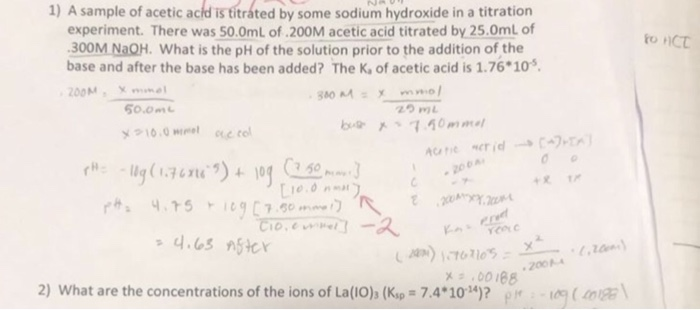1) A sample of acetic acld is titrated by some sodium hydroxide in a titration experiment. There was 50.0mL of .200M acetic acid titrated by 25.0mL of 300M NaOH. What is the pH of the solution prior to the addition of the base and after the base has been added? The K, of acetic acid is 1.76*10 200M HICT 0.0m 2つmL eo 200 2) what are the concentrations of the ions of La(IO)3 (Ksp=7.4*1014)? PM-1 (a, \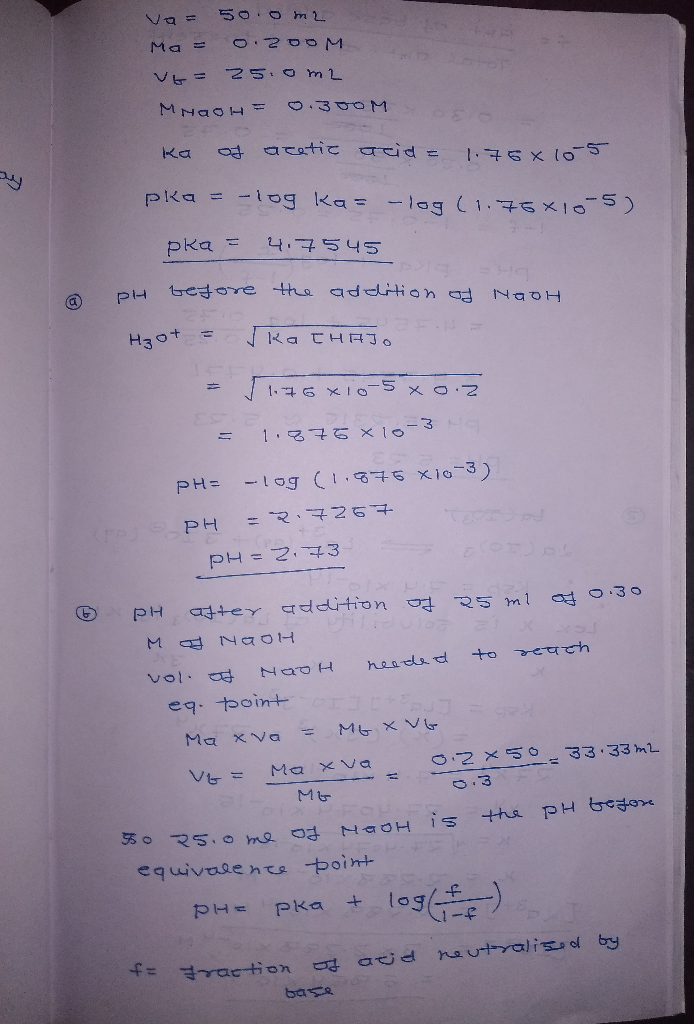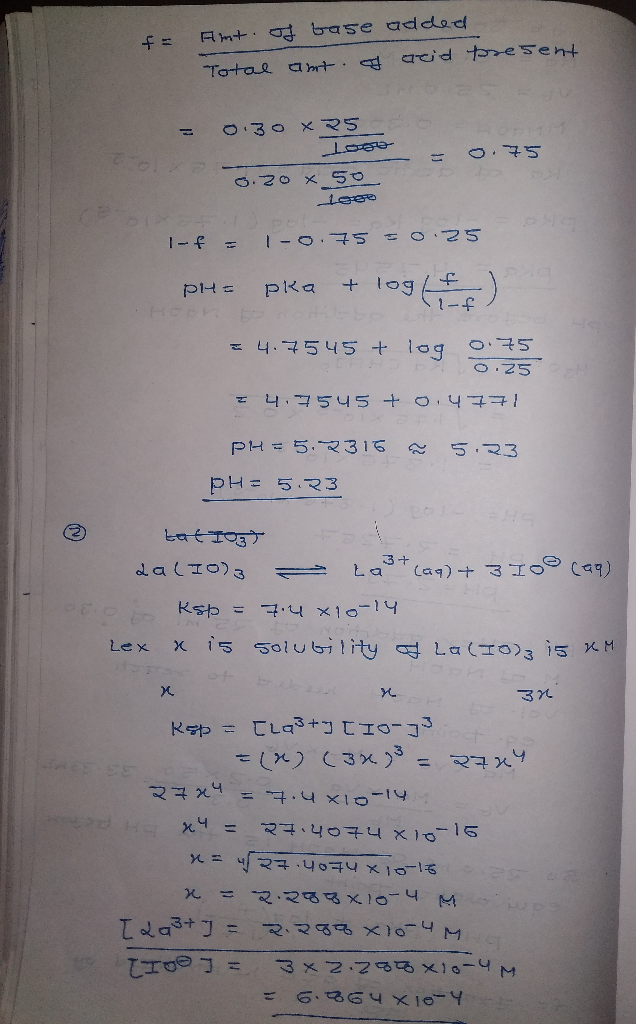#### Earn Coin

Coins can be redeemed for fabulous gifts.

Similar Homework Help Questions
• ### 1. During a titration experiment, a student titrated 25.00 mL of a 0.100 M sodium hydroxide...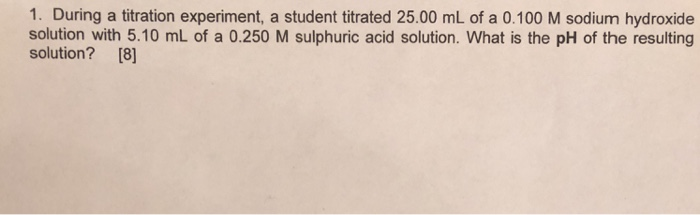1. During a titration experiment, a student titrated 25.00 mL of a 0.100 M sodium hydroxide solution with 5.10 mL of a 0.250 M sulphuric acid solution. What is the pH of the resulting solution? 

• ### Refer to the titration curve above for 0.500 M acetic acid titrated with hydroxide ions. When...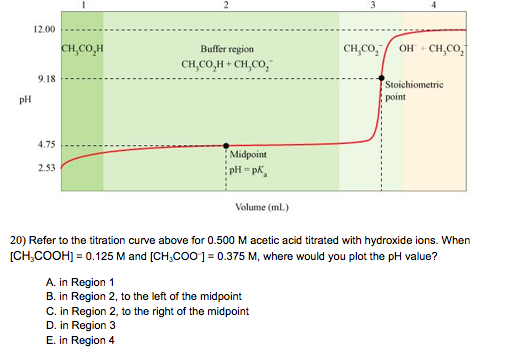Refer to the titration curve above for 0.500 M acetic acid titrated with hydroxide ions. When [CH3COOH] = 0.125 M and [CH3COO-] = 0.375 M, where would you plot the pH value? in Region 1 in Region 2. to the left of the midpoint in Region 2. to the right of the midpoint in Region 3 in Region 4

• ### In the titration of acetic acid (CH3COOH) and sodium hydroxide (NaOH), what are the major sepcies...

In the titration of acetic acid (CH3COOH) and sodium hydroxide (NaOH), what are the major sepcies present at the following points? a) Before any NaOH is added (at the start of the titration). b) In the buffer region. Are there any spectator ions? c) At equivalence point. What is equivalent at this point? What has been used up? Are there any spectator ions? d) Beyond equivalence point. What has been added since the equivalence point? Has anything reacted further since...

• ### 1) A 34.2 mL sample of a 0.590 M aqueous acetic acid solution is titrated with...

1) A 34.2 mL sample of a 0.590 M aqueous acetic acid solution is titrated with a 0.203 M aqueous potassium hydroxide solution. What is the pH after 37.1 mL of base have been added? 2) What is the pH at the equivalence point in the titration of a 25.5 mL sample of a 0.351 M aqueous hypochlorous acid solution with a 0.433 M aqueous barium hydroxide solution?

• ### U Experiment 17B: Acid-Base Titration Report Data Table Trial 1 Trial 2 T Trial 3 Volume...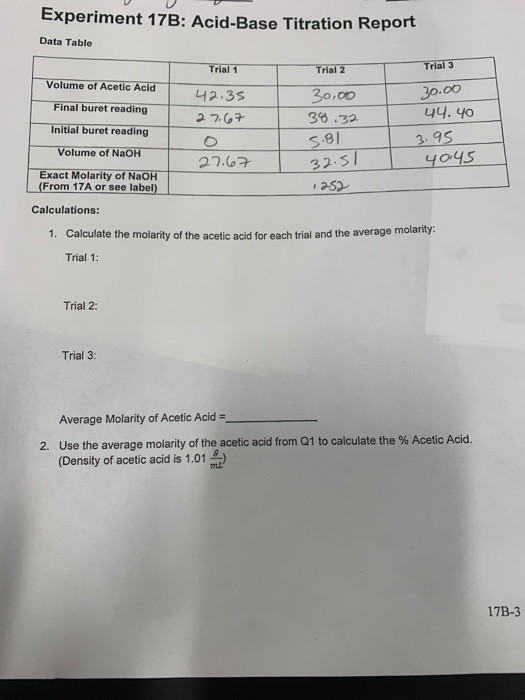U Experiment 17B: Acid-Base Titration Report Data Table Trial 1 Trial 2 T Trial 3 Volume of Acetic Acid Final buret reading 42.35 27.67 Initial buret reading 30.00 38.32 5.81 32.51 30.00 44.40 3.95 40.45 Volume of NaOH 27.67 Exact Molarity of NaOH (From 17A or see label) 1252 Calculations: 1. Calculate the molarity of the acetic acid for each trial and the average molarity Trial 1: Trial 2: Trial 3: Average Molarity of Acetic Acid = 2. Use the...

• ### 1. A weak acid (benzoic acid) is titrated with a strong base such as sodium hydroxide....

1. A weak acid (benzoic acid) is titrated with a strong base such as sodium hydroxide. Determine the pH at the half equivalence point of the titration. The Ka of the weak acid is 6.3 x 10-5. 2.Calculate the pH of a buffer made from 0.6 M HNO2 and 0.5 M NaNO2. Ka = 4.5 x 10-5 pKa = 4.3 Answer with one digit after the decimal place (e.g. 2.1) Hint: HNO2 is a weak acid. NaNO2 forms the conjugate...

• ### i need all answers Data Table 1: Titration of Vinegar with Sodium Hydroxide Vinegar Sample 1...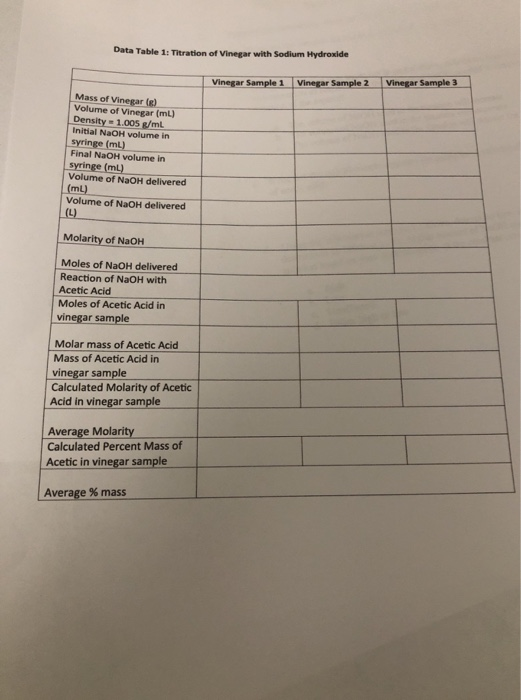i need all answers Data Table 1: Titration of Vinegar with Sodium Hydroxide Vinegar Sample 1 Vinegar Sample 2 Vinegar Sample 3 Mass of Vinegar (6) Volume of Vinegar (ml) Density - 1.005 g/ml Initial NaOH volume in syringe (ml) Final NaOH volume in syringe (ml) Volume of NaOH delivered (mL) Volume of NaOH delivered (L) Molarity of NaOH Moles of NaOH delivered Reaction of NaOH with Acetic Acid Moles of Acetic Acid in vinegar sample Molar mass of Acetic...

• ### 14 13 1. A 25.00 mL sample of 0.100 M acetic acid is titrated by adding...14 13 1. A 25.00 mL sample of 0.100 M acetic acid is titrated by adding 0.100 M sodium hydroxide. What is the pH of the acetic acid solution before the titration begins? 12 11 10 a. What is the pH after 12.50 mL of NaOH have been added? 9 b. What is the pH after 25.00 mL of NaOH have been added? 8 7 6 5 c. What is the pH after 37.50 mL of NaOH have been added?...

• ### In a titration, 25 mL of 0.10 M weak diprotic acid solution was titrated by 0.10 M sodium hydroxide, NaOH, and produ...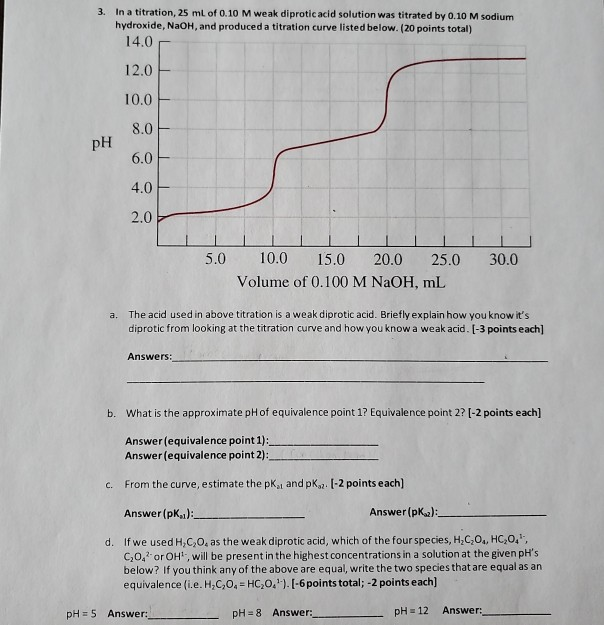In a titration, 25 mL of 0.10 M weak diprotic acid solution was titrated by 0.10 M sodium hydroxide, NaOH, and produced a titration curve listed below. (20 points total) 14,0 3. 12.0 10.0 8.0 pH 6.0 4.0 2.0 10.0 5.0 20.0 30.0 15.0 25.0 Volume of 0.100 M NaOH, mL The acid used in above titration is a weak diprotic acid. Briefly explain how you know it's diprotic from looking at the titration curve and how you know a...

• ### Experiment Quantitative Titration - Part 1: Standardization of Sodium Hydroxide Solution Concentration of HCL standard solution...

Experiment Quantitative Titration - Part 1: Standardization of Sodium Hydroxide Solution Concentration of HCL standard solution / mol L^-1 = 0.09745 Volume of HCL solution / mL = 25 Indicator: Bromothymol Blue Average Volume of NaOH / mL = 24.35 Please find concentration of NaOH / mol^-1 Part 2 - Determination of the concentration of acetic acid Volume of Acedic Acid Solution / mL = 10 Indicator: Phenolphthalein Average Volume of NaOH / mL = 30.94 Please find the concentration...

Free Homework App